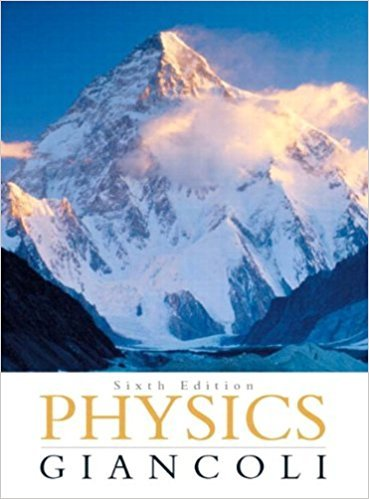×
×

# Solved: A hypothetical planet has a radius 1.5 times thatISBN: 9780321569837 99

## Solution for problem 31P Chapter 5

Physics: Principles with Applications | 6th Edition

• Textbook Solutions
• 2901 Step-by-step solutions solved by professors and subject experts
• Get 24/7 help from StudySoup virtual teaching assistantsPhysics: Principles with Applications | 6th Edition

4 5 1 294 Reviews
28
3
Problem 31P

A hypothetical planet has a radius 1.5 times that of Earth, but has the same mass. What is the acceleration due to gravity near its surface?

Step-by-Step Solution:

Solution 31P

Step 1 of 3:

In this question we need to find acceleration due to gravity of a hypothetical planet

Radius of EarthStep 2 of 3:

We can find gravitational force using relationComparing gravity of earth with other planetHence we have ratio of gravitational force on Earth to another planet asStep 3 of 3

##### ISBN: 9780321569837

This textbook survival guide was created for the textbook: Physics: Principles with Applications, edition: 6. Since the solution to 31P from 5 chapter was answered, more than 303 students have viewed the full step-by-step answer. Physics: Principles with Applications was written by and is associated to the ISBN: 9780321569837. This full solution covers the following key subjects: acceleration, due, EARTH, gravity, hypothetical. This expansive textbook survival guide covers 33 chapters, and 4141 solutions. The answer to “A hypothetical planet has a radius 1.5 times that of Earth, but has the same mass. What is the acceleration due to gravity near its surface?” is broken down into a number of easy to follow steps, and 26 words. The full step-by-step solution to problem: 31P from chapter: 5 was answered by , our top Physics solution expert on 09/09/17, 04:43AM.

Unlock Textbook Solution